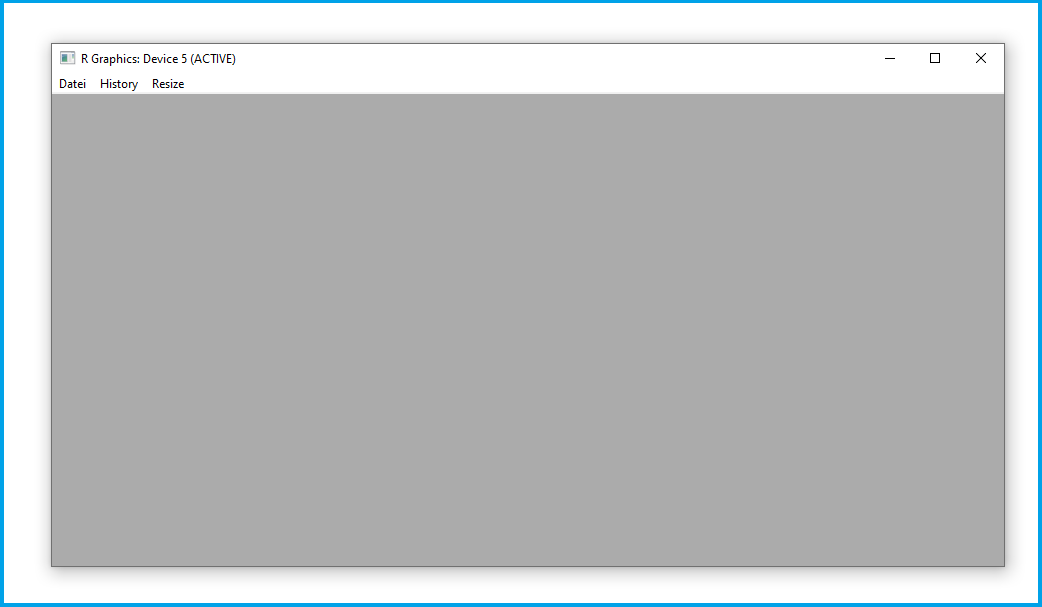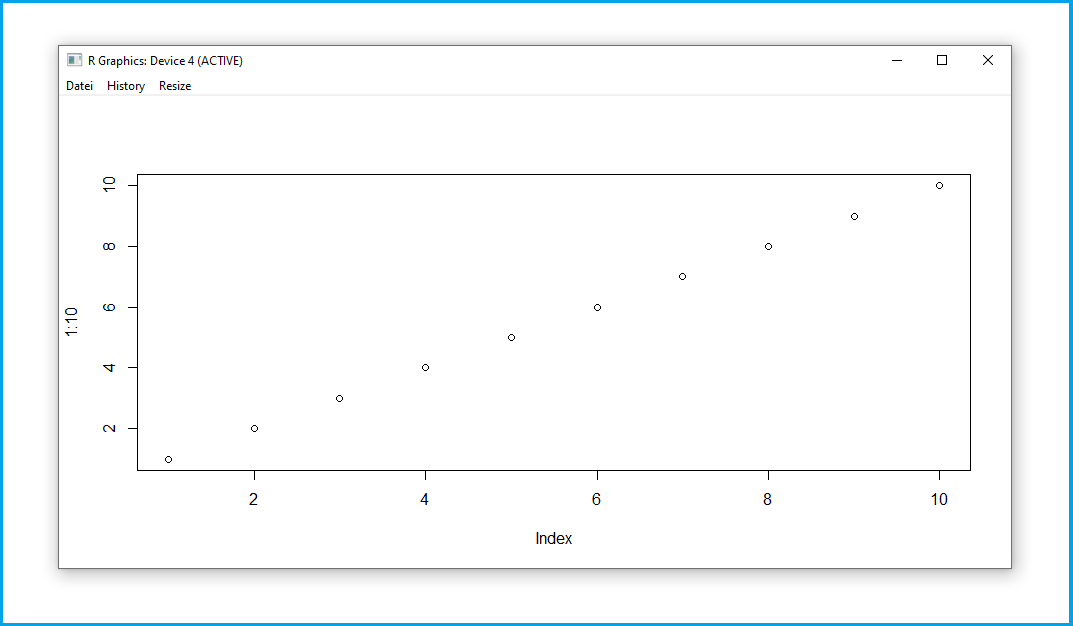# x11 Function in R (Example) | Start Graphics Device Driver for X Window System

In this post, I’ll show how to start a graphics device driver for the X Window System using the x11() function in R programming.

You’re here for the answer, so let’s get straight to the examples…

## Example 1: Basic Application of x11() Function

In this example, I’ll explain how to apply the x11 function to create a new window for the X Window System graphics device driver.

For this, we can use the x11 function as shown below. Within the x11 function, we can specify the width and height of our window:

```x11(width = 10,     # Apply x11 function
height = 5)```After executing the previous R code, the empty plotting window shown in Figure has been created.

## Example 2: Draw Plot after Starting Graphics Device Driver Using x11() Function

The following R code demonstrates how to draw a graph after starting the X Window System graphics device driver in R.

For this, we simply have to execute the code for the creation of a graph after running the x11 function:

```x11(width = 10,     # Apply x11 function
height = 5)
plot(1:10)          # Draw plot```Figure 2 shows the output of the previous R code. We have drawn a scatterplot in a new X Window System graphics device driver window.

## Video, Further Resources & Summary

Would you like to know more about the usage of the x11() function? Then you may want to have a look at the following video on my YouTube channel. I’m explaining the R programming code of this article in the video tutorial:

Furthermore, you may want to have a look at the other tutorials on https://statisticsglobe.com/:

In this R programming tutorial you have learned how to apply the x11() function.

Please note that the previous R code may lead to different results depending on the operating system you are using. In my case, I’m using a Windows machine. However, in case you are using other devices based on Mac or Linux, you may face the error messageError in x11() : X11 is not available“.

If you have further questions, don’t hesitate to tell me about it in the comments.

Subscribe to the Statistics Globe Newsletter